Win up to 100% scholarship on Aakash BYJU'S JEE/NEET courses with ABNAT Win up to 100% scholarship on Aakash BYJU'S JEE/NEET courses with ABNAT

# JEE Advanced 2020 Chemistry Paper 1

JEE Advanced 2020 question paper for Chemistry (Paper 1) is provided here along with solutions that have been created by our subject experts. Chemistry Paper 1 section had a total of 18 questions, 66 marks respectively. Questions were framed from the different sections. Students can have a glance at the question paper and get a better understanding of the question types, difficulty level, marking scheme.
They can further take their time to solve these questions and test their preparation level. The solutions that are provided will help them find accurate answers and ultimately have an effective preparation for the entrance exam. Each question comes with a brief explanation that justifies why it is the correct answer. Students can access this set of questions and answers freely and it is also available in a downloadable PDF format at the bottom.
Paper 1 – Chemistry

Question 1. If the distribution of molecular speeds of gas is as per the figure shown below, then the ratio of the most probable, the average, and the root mean square speeds, respectively, is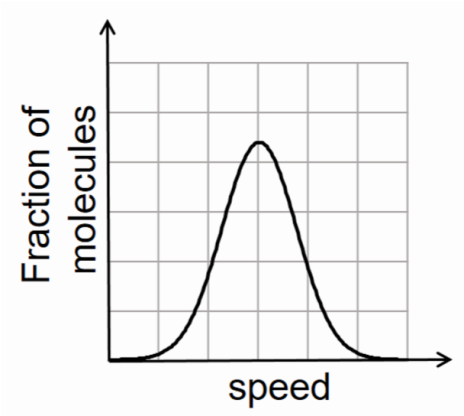a) 1 : 1 : 1
b) 1 : 1 : 1.224
c) 1 : 1.128 : 1.224
d) 1 : 1.128 : 1

As a symmetrical graph is given, the average value of speed will be the middle symmetrical speed which is also the most probable speed.

When we are calculating the rms speed we have to take the average of square of speeds and its root. In square of speed, higher speeds will give more contribution so the value will be more than the average value.

Question 2. Which of the following liberates O2 upon hydrolysis?

a) Pb3O4
b) KO2
c) Na2O2
d) Li2O2

a) Pb3O4 + H2O → No reaction (due to insoluble in water)

b) Superoxides liberate oxygen with water.

2KO2 + 2H2O → 2KOH + H2O2 + O2

c) Na2O2 + 2H2O → 2NaOH + H2O2

d) Li2O2 + 2H2O → 2LiOH + H2O2

Question 3. A colourless aqueous solution contains nitrates of two metals, X and Y. When it was added to an aqueous solution of NaCl, a white precipitate was formed. This precipitate was found to be partially soluble in hot water to give a residue P and a solution Q. The residue P was soluble in aq. NH3 and also in excess sodium thiosulfate. The hot solution Q gave a yellow precipitate with KI. The metals X and Y, respectively, are

a) Ag and Pb
b) Ag and Cd
c) Cd and Pb
d) Cd and Zn

x = Ag; y = Pb; P = AgCl; Q = PbCl2

(1) AgNO3 + NaCl→AgCl↓ + NaNO3

(x) (P)

(2) AgCl(s)↓ + 2NH3 → [Ag(NH3)2]+ Cl

(P) (soluble complex)

(3) AgCl + 2Na2S2O→ Na3[Ag(S2O3)] + NaCl

(residue-p) (excess) (soluble complex)

(4) Pb(NO3)2+ 2NaCl → PbCl2↓+ 2NaNO3

(y) white ppt(Q)

(soluble in hot water)

(5) PbCl2 + 2KI → PbI2 + 2KCl

(hot solution) yellow ppt

Q

Question 4. Newman projections P, Q, R and S are shown below: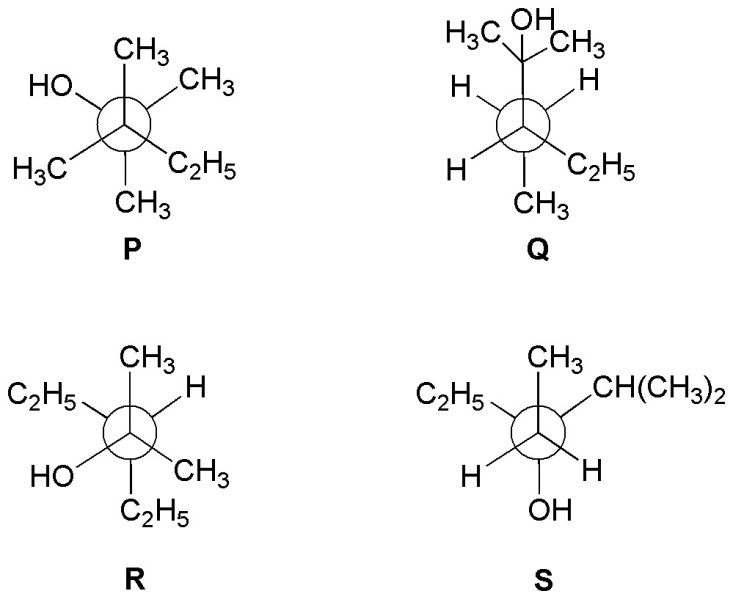Which one of the following options represents identical molecules?

a) P and Q
b) Q and S
c) Q and R
d) R and S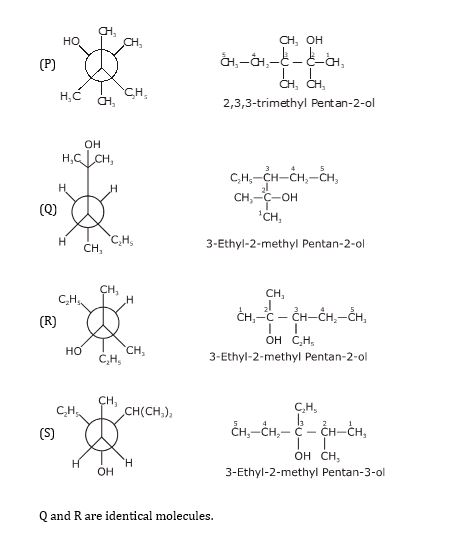Question 5. Which one of the following structures has the IUPAC name 3-ethynyl-2-hydroxy-4-methylhex-3-en-5-ynoic acid?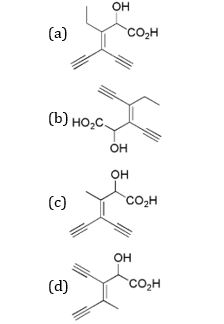3-ethynyl-2-hydroxy-4-methylhex-3-en-5-ynoic acid

Question 6. The Fischer projection of D-erythrose is shown below.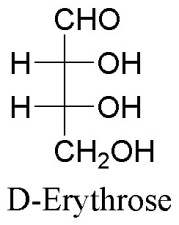D-Erythrose and its isomers are listed as P, Q, R, and S in Column-I. Choose the correct relationship of P, Q, R, and S with D-erythrose from Column II.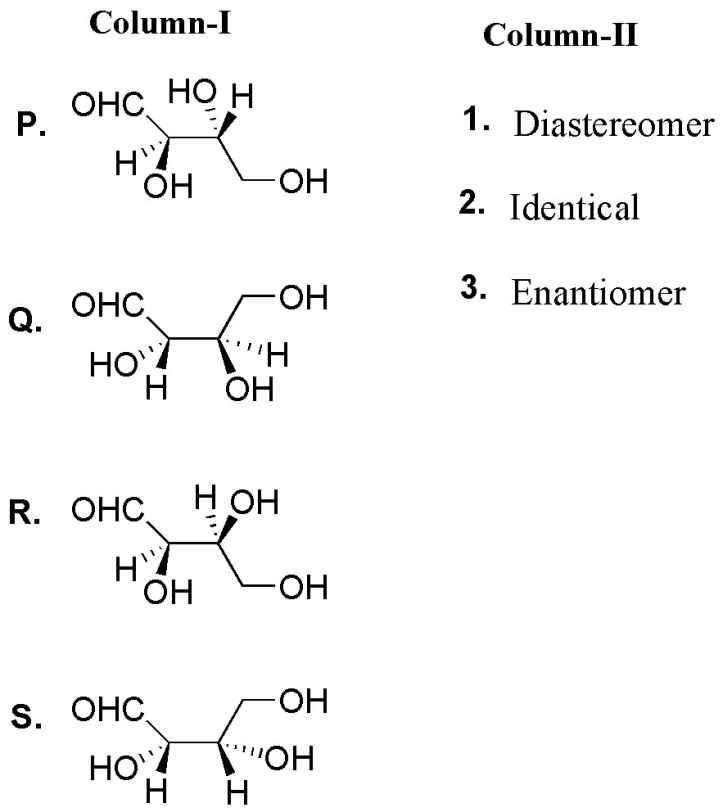a) P→2, Q→3, R→2, S→2
b) P→3, Q→1, R→1, S→2
c) P→2, Q→1, R→1, S→3
d) P→2, Q→3, R→3, S→1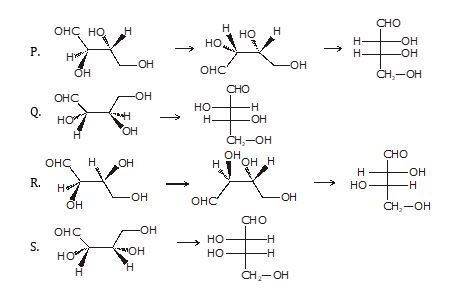Question 7. In thermodynamics, the 𝑃 − 𝑉 work done is given by

$$\begin{array}{l}w = -\int dVP_{ext}\end{array}$$
. For a system undergoing a particular process, the work done is,

$$\begin{array}{l}w = -\int dV \left (\frac{RT}{V-b}-\frac{a}{V^{2}} \right )\end{array}$$

This equation is applicable to a

a) System that satisfies the van der Waals equation of state.
b) Process that is reversible and isothermal.
c) Process that is reversible and adiabatic.
d) Process that is irreversible and at constant pressure.

$$\begin{array}{l}w = -\int P_{ext.} dV\end{array}$$

From van der waal equation of state for one mole gas.

$$\begin{array}{l}\left ( P+\frac{a}{V^{2}} \right )(v-b) = RT\end{array}$$
$$\begin{array}{l}P = \left [ \frac{RT}{V-b}-\frac{a}{V^{2}} \right ]\end{array}$$

For reversible process

Pext. = Pgas

$$\begin{array}{l}W = -\int \left ( \frac{RT}{V-b}-\frac{a}{V^{2}} \right )dv\end{array}$$

So, process is not applicable only for irreversible process.

Hence Ans, a, b, c

Question 8. With respect to the compounds I-V, choose the correct statement(s).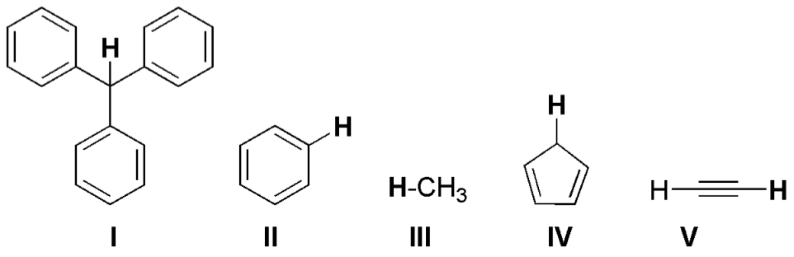a) The acidity of compound I is due to delocalization in the conjugate base.
b) The conjugate base of compound IV is aromatic.
c) Compound II becomes more acidic, when it has a -NO2 substituent.
d) The acidity of compounds follows the order I >IV>V>II>III.

a) is a conjugate base of compound I Which is stabilized by delocalization or resonance.

b) is a conjugate base of, which is an aromatic compound.

c) –NO2 group is a strong electron-withdrawing group, which increases the acidic strength of compound II.

d) The order of acidic strength.

Question 9. In the reaction scheme shown below, Q, R, and S are the major products.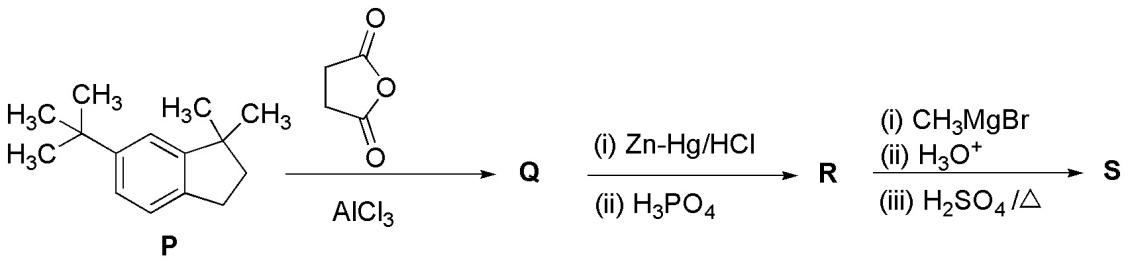The correct structure of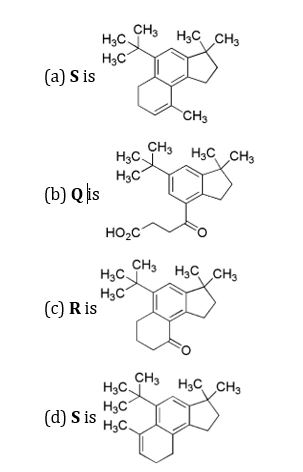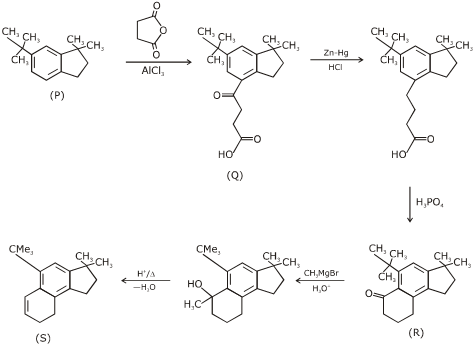Question 10. Choose the correct statement(s) among the following:

a) [FeCl4] has tetrahedral geometry.
b) [Co(en)(NH3)2Cl2]+has 2 geometrical isomers.
c) [FeCl4] has higher spin-only magnetic moment than [Co(en)(NH3)2Cl2]+.
d) The cobalt ion in [Co(en)(NH3)2Cl2]+ has s𝑝3𝑑2 hybridization.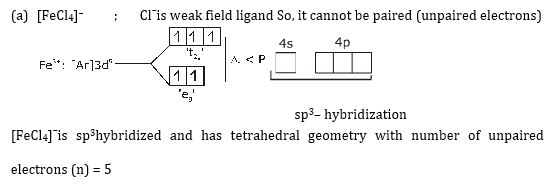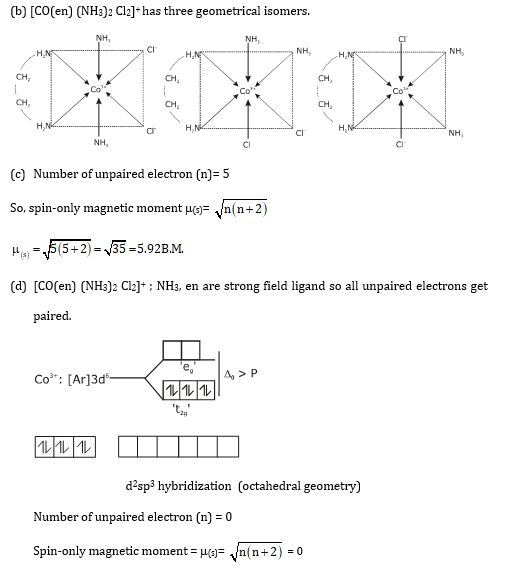Question 11. With respect to hypochlorite, chlorate and perchlorate ions, choose the correct statement(s).

a) The hypochlorite ion is the strongest conjugate base.
b) The molecular shape of only chlorate ion is influenced by the lone pair of electrons of Cl.
c) The hypochlorite and chlorate ions disproportionate to give rise to an identical set of ions.
d) The hypochlorite ion oxidizes the sulfite ion.

Hypochlorite ion → ClO

Chlorate ion → ClO3

Perchlorate ion → ClO4

Acidic nature → HClO< HClO3< HClO4

Conjugate Base order → ClO> ClO3> ClO4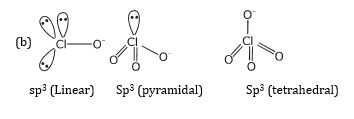In chlorate ion bond angle changes due to presence of lone pair on chlorine atom while hypochlorite ion is linear and perchlorate ion is tetrahedral and there is no effect of lone pair on hypochlorite ion.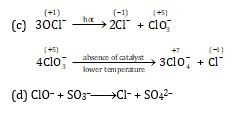Question 12. The cubic unit cell structure of a compound containing cation M and anion X is shown below. When compared to the anion, the cation has a smaller ionic radius. Choose the correct statement(s).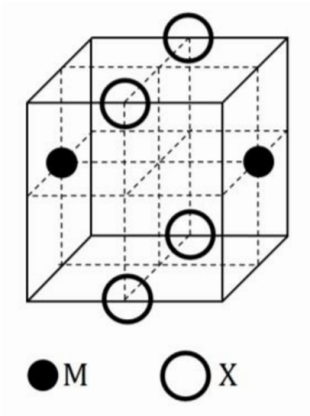a) The empirical formula of the compound is MX.
b) The cation M and anion X have different coordination geometries.
c) The ratio of M-X bond length to the cubic unit cell edge length is 0.866.
d) The ratio of the ionic radii of cation M to anion X is 0.414.

According to the given diagram, the structure seems to be B.C.C.

(1) Empirical formula → MX

(2) C. No. of ‘M’ ion = 8 [same co-ordination Geometry]

C. No. of ‘X’ ion = 8 [same co-ordination Geometry]

(3) M–X Bond Length =

$$\begin{array}{l}\sqrt{\left(\frac{a}{2}\right)^{2}+\left (\frac{a}{\sqrt{2}}\right)^{2}}\end{array}$$

M–X Bond Length =

$$\begin{array}{l}a = \sqrt{\frac{1}{4}+\frac{1}{2}}\end{array}$$
$$\begin{array}{l}\frac{M-Xbondlength}{a (edgelength)}=\frac{\sqrt{3}}{2} = 0.866\end{array}$$

(4) As it is B.C.C.

$$\begin{array}{l}r_{x-} + r_{m-} =\sqrt{\frac{3}{2}a}\end{array}$$
$$\begin{array}{l}0.732\leq \frac{r_{m+}}{r_{x-}}< 1\end{array}$$

Ans. A,C

Question 13. 5.00 mL of 0.10 M oxalic acid solution taken in a conical flask is titrated against NaOH from a burette using phenolphthalein indicator. The volume of NaOH required for the appearance of permanent faint pink color is tabulated below for five experiments. What is the concentration, in molarity, of the NaOH solution?

 Exp. No. Vol. of NaOH (mL) 1 12.5 2 10.5 3 9.0 4 9.0 5 9.0

H2C2O4 + 2NaOH → Na2C2O4 + 2H2O

5ml M

0.1 M

By law of equivalence

Number of eq. of NaOH = Number of eq. of oxalic acid

$$\begin{array}{l}M = \left [ NaOH \right ] = \frac{\left [ H_{2}C_{2}O_{2} \right ]X vol. X 2}{vol.of NaOH}\end{array}$$
$$\begin{array}{l}\left [ NaOH \right ]_{1} = \frac{5\times 0.1 \times 2}{12.5} = \frac{1}{12.5}\end{array}$$
$$\begin{array}{l}\left [ NaOH \right ]_{2} = \frac{5\times 0.1 \times 2}{10.5} = \frac{1}{10.5}\end{array}$$
$$\begin{array}{l}\left [ NaOH \right ]_{3} = \frac{5\times 0.1 \times 2}{9} = \frac{1}{9} = \left [ NaOH \right ]_{4} = \left [ NaOH \right ]_{5}\end{array}$$
$$\begin{array}{l}\left [ NaOH \right] Final Result =\frac{\frac{1}{12.5}+\frac{1}{10.5}+\frac{1}{9}\times 3}{5}\end{array}$$
$$\begin{array}{l}\left [NaOH\right]Final Result =\frac{\frac{2}{25}+\frac{2}{21}+\frac{1}{3}}{5}\end{array}$$
$$\begin{array}{l}\left [ NaOH \right ] Final Result =\frac{0.08 + 0.095+0.333}{5}\end{array}$$

= 0.102 ≃ 0.11 Ans.

Question 14. Consider the reaction A ⇌ B at 1000 K. At time 𝑡’, the temperature of the system was increased to 2000 K and the system was allowed to reach equilibrium. Throughout this experiment, the partial pressure of A was maintained at 1 bar. Given below is the plot of the partial pressure of B with time.

What is the ratio of the standard Gibbs energy of the reaction at 1000 K to that at 2000 K?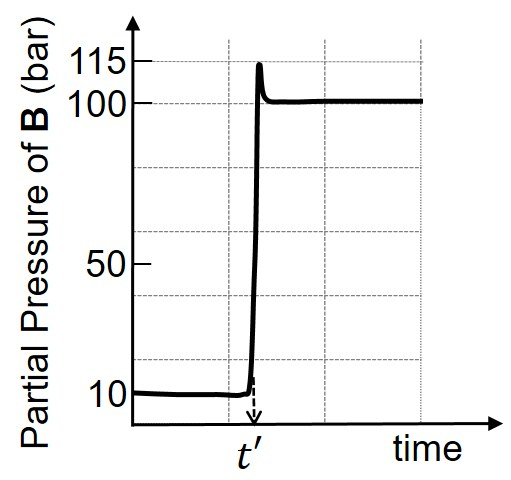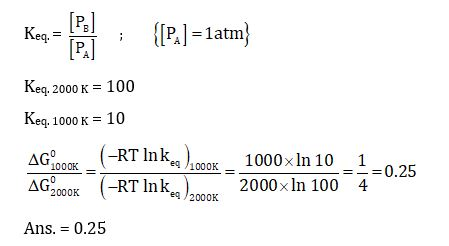Question 15. Consider a 70% efficient hydrogen-oxygen fuel cell working under standard conditions at 1 bar and 298 K. Its cell reaction is

H2(g) + ½ O2(g) → H2O(l)

The work derived from the cell on the consumption of 1.0 × 10−3mol of H2(𝑔) is used to compress 1.00 mol of a monoatomic ideal gas in a thermally insulated container. What is the change in the temperature (in K) of the ideal gas?

The standard reduction potentials for the two half-cells are given below.

O2(𝑔) + 4H+(𝑎𝑞) + 4𝑒→ 2 H2O (𝑙), 𝐸0 = 1.23 V,

2H+(𝑎𝑞) + 2𝑒→ H2 (𝑔), 𝐸0 = 0.00 V

Use 𝐹 = 96500 C mol−1, R = 8.314 J mol−1 K−1.

Eocell = 1.23 volt

∆G0 = –nF Eocell {-2 x 96500 x 1.23} J

Since, Work derived from this fuel cell = 70 / 100 x (- ∆Gocell) x 1.0 x 10-3 = x J

Since insulated vessel, hence q = 0

From equation, for monoatomic gas,

w = ∆U ⇒ x = nCV,m∆T ;

$$\begin{array}{l}\left \{ C_{V,m}= \frac{3R}{2} \right \}\end{array}$$

Or

70 / 100 x 2 X 96500 X 1.23 X 10-3 = 1 X 3/2 X 8.314 X ∆T

∆T = 13.32

Question 16. Aluminum reacts with sulfuric acid to form aluminum sulfate and hydrogen. What is the volume of hydrogen gas in liters (L) produced at 300 K and 1.0 atm pressure, when 5.4 g of aluminum and 50.0 mL of 5.0 M sulfuric acid are combined for the reaction?

(Use molar mass of aluminum as 27.0 g mol−1, 𝑅 = 0.082 atm L mol−1 K−1)

2Al + 3H2SO4 → Al2(SO4)3 + 3H2

Mole of Al taken = 5.4 / 27 = 0.2

Mole of H2SO4 taken = 50 X 5 / 1000 = 0.25

As 0.2 / 2 > 0.25 / 3, H2SO4 is limiting reagent

Now, moles of H2 formed = 3 / 3 X 0.25 = 0.25

Therefore Volume = 0.25 x 0.082 x 300 / 1 = 24.6 / 4 = 6.15 L

Question 17.

$$\begin{array}{l}_{92}^{238}\textrm{u}\end{array}$$
is known to undergo radioactive decay to form
$$\begin{array}{l}_{82}^{206}\textrm{Pb}\end{array}$$
by emitting alpha and beta particles. A rock initially contained 68 × 10−6 g of
$$\begin{array}{l}_{92}^{238}\textrm{u}\end{array}$$
. If the number of alpha particles that it would emit during its radioactive decay of
$$\begin{array}{l}_{92}^{238}\textrm{u}\end{array}$$
to
$$\begin{array}{l}_{82}^{206}\textrm{Pb}\end{array}$$
in three half-lives is
𝑍 × 1018, then what is the value of𝑍?

$$\begin{array}{l}_{92}U^{238}\rightarrow _{82}Pb^{206} + 8_{2}He^{4}+6_{-1}\beta ^{0}\left ( antineutrino \right )\end{array}$$
$$\begin{array}{l}Initial mole of u^{238} = \left [ \frac{68\times 10^{-6}}{238} \right ] = x\end{array}$$

Mole of U238 decayed in three half-lives = 7/ 8 x

In decay from U238 to Pb206, each U238 atom decays and produces 8 α-particles and hence, total number of α-particles emitted out =

$$\begin{array}{l}\left ( \frac{7}{8}x \right )\times 8\times N_{A}\end{array}$$

=

$$\begin{array}{l}\frac{7}{8}\times \frac{68 \times 10^{-6}}{238} \times 8\times 6.022\times 10^{23}\end{array}$$

= 1.2046 × 1018

= 1.21

Question 18. In the following reaction, compound Q is obtained from compound P via an ionic intermediate.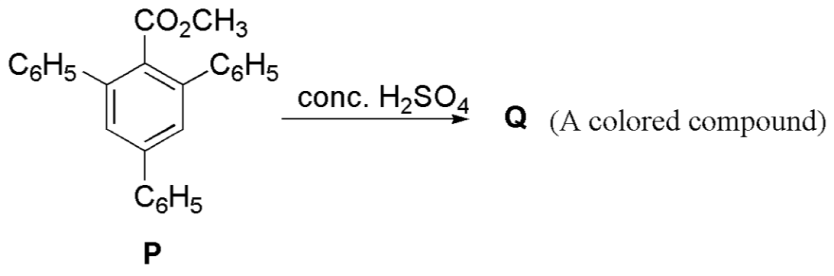What is the degree of unsaturation of Q?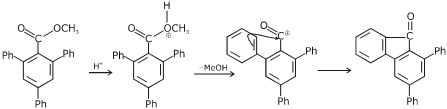The total degree of unsaturation = 18

## Video Lessons – Paper 1 Chemistry